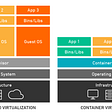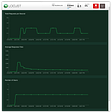# Augmenting RSDF output from SAP BW

## 1. Import Libraries:

`import osimport reimport pyhdbimport pandas as pdimport xxx_dbconfig as configfrom lxml import htmlfrom bs4 import BeautifulSoup`
`hanadb = {'host': "xxx.xxx.xxx.xxx",         'port': *****,         'user': "********",         'passwd': "*********"}`

## 2. Function to build list of HTML files

`def get_inputhtml(base_path, print_on):    input_path = base_path + '/input_html/'    folder = os.fsencode(input_path)    filenames = []    os.chdir(input_path)    # loop through 'input_html' folder for all HTML files    for file in os.listdir(folder):        filename = os.fsdecode(file)        if filename.endswith( ('.HTML') ):            filenames.append(filename)    filenames.sort()    return(filenames)`

## 3. Function to extract the soup

`def get_soup(web_template, base_path, print_on): #read an HTML file and construct the soup object    input_path = base_path + '/input_html/'    os.chdir(input_path)    f = open(web_template)    soup = BeautifulSoup(f, 'html5lib')    f.close()    if print_on == 'X':        print('Finished getting soup ...')    return(soup)`

## 4. Function to find Transformations in the soup

`def get_transformations(soup, print_on): #scan soup object and collect unique transformations    # find all transformations in html doc    trfms = []    for element in soup.find_all(text=re.compile('Transformation:')):        parentTable = element.findParent('table')        technm_cell = parentTable.find(text=re.compile('Technical Name:'))        trfm = technm_cell.findNext('td')        trfms.append(trfm.text)    if print_on == 'X':        print('Finished getting transformations ...')    return(trfms)`

## 5. Function to clean up the soup

`def clean_soup(soup, print_on, rem_extra_detail): #clean up various links    # remove extra detail ['Required Objects', 'ADSO Flags', 'Relays data to', 'Receives data from', 'Used by',  'Data Transfer Process']     if rem_extra_detail == 'X':        extra_detail_elements = ['Required Objects', 'ADSO Flags', 'Relays data to', 'Receives data from', 'Used by',  'Data Transfer Process']         for extra_detail in extra_detail_elements:            for block in soup.find_all(text=re.compile(extra_detail)):                if extra_detail == 'Data Transfer Process':                    parentTable = block.findParent('table')                    parentTable.decompose()                else:                    parentTbody = block.findParent('tbody')                    parentTbody.decompose()            if print_on == 'X':                print('Removed blocks containing: ' + extra_detail)    # remove broken links from the first 'Reqired Objects' table (if not already removed above)    indx = 0    for broken_links in soup.find_all(text=re.compile('Required Objects')):        if indx == 0:            bl_parentTbody = broken_links.findParent('tbody')            for bl_match in bl_parentTbody.find_all('a'):                bl_match.replaceWithChildren()        indx += 1    # remove spans that contain 'FORMULA'    for formula_spans in soup.find_all(text=re.compile('Formula:')):        parentSpan = formula_spans.findParent('span')        parentSpan.decompose()    # repair/reformat FORMULA links    invalid_tags = ['a', 'b', 'u']    for tag in invalid_tags:        for formula_links in soup.find_all(text=re.compile('FORMULA')):            parentTd = formula_links.findParent('td')            for match in parentTd.findAll(tag):                match.replaceWithChildren()    if print_on == 'X':        print('Cleaned the soup ...')    return(soup)`

## 6. Function to retrive the missing detail from SAP BW

`def get_data(trfms, print_on): #get active_formulas and active_tranfields    # load data from HANA db    connection = pyhdb.connect(host=config.hanadb['host'],                                port=config.hanadb['port'],                               user=config.hanadb['user'],                                password=config.hanadb['passwd'])    hana_list = str(trfms).replace('[', '(').replace(']', ')')    cursor = connection.cursor()    #Deliberately not relying on a single view to avoid HANA dependencies    #Get active formulas for all transformations in the document    where_clause = '(("TRANID" in ' + hana_list + ') AND ("OBJVERS" = \'A\') AND ( "KIND" = \'FORMULA\') )'    stmnt = 'SELECT * from SAPBWH.RSTRANSTEPROUT WHERE ' + where_clause    cursor.execute(stmnt)    active_formulas = cursor.fetchall()    #Get ibjs for rule steps above    where_clause = '(("OBJVERS" = \'A\') AND ("TRANID" in ' + hana_list + ') AND ("PARAMTYPE" = \'1\'))'    stmnt = 'SELECT * FROM "SAPBWH"."RSTRANFIELD" WHERE ' + where_clause     cursor.execute(stmnt)    active_tranfields = cursor.fetchall()    #Get rule step texts (used for formulas)    where_clause = '( ("LANGU" = \'EN\') AND ("TRANID" in ' + hana_list + ') AND ("OBJVERS" = \'A\') )'    stmnt = 'SELECT * FROM "SAPBWH"."RSTRANRULET" WHERE ' + where_clause     cursor.execute(stmnt)    active_trantexts = cursor.fetchall()    cursor.close()        #!!*** todo: get Descriptions ***!!    #for tran_step in active_formulas:    #active_formulas_wtxts = [ft for ft in active_trantexts if ft == tran_step[]]    #!!*** ******************* ***!!        if print_on == 'X':        print('Retrieved data from HANA ...')    return(active_formulas, active_tranfields)`

## 7. Function to inject the Formula detail into the HTML

`def inject_formulas(trfms, soup, active_formulas, active_tranfields, print_on):    # loop over transformations and link formula code, and insert    for trfmation in trfms:        if print_on == 'X':            print('++++++++++++++++++++')            print('Trfmtn: ' + trfmation)        formula_steps = [sfs for sfs in active_formulas if sfs == trfmation]        for single_formula_step in formula_steps:            #formula_descr = 'Description: ' +             formula_cd = 'Formula: ' + str(single_formula_step)            single_trfm_rules = [y for y in active_tranfields if y == trfmation]            single_step = [y for y in single_trfm_rules if y == single_formula_step]            iobj_id = single_step            if print_on == 'X':                print('IObj: ' + iobj_id)                print(formula_cd)                print('____________________')            span_txt = formula_cd            span_id = 'formula_' + iobj_id            new_span = soup.new_tag('div', id=span_id)                  new_span.string = str(span_txt)            new_br = soup.new_tag('br')            new_span.insert(0, new_br)            for element2 in soup.find_all(text=re.compile('Transformation:')):                parentTable2 = element2.findParent('table')                curr_trfm_cell = parentTable2.find(text=re.compile('Technical Name:'))                curr_trfm_id = curr_trfm_cell.findNext('td').text                if curr_trfm_id == trfmation:                    group_table = parentTable2.find(text=re.compile('Group')).find_parent('table')                    iobj_element = group_table.find(text=re.compile(str(iobj_id)))                    parentTbody = iobj_element.find_parent('tbody')                    parentTbody.find_all("span")[-2].append(new_span)    if print_on == 'X':        print('Finished injecting Formulas ...')    return(soup)`

## 8. Function to write the corrected HTML to file

`def write_output(soup, base_path, print_on):    # output modified html    html_output_path = base_path + '/output_html/'    os.chdir(html_output_path)    html = soup.prettify("latin-1")    input_fileName,fileType=web_template.split('.')    output_filename = input_fileName + "_output." + fileType    if print_on == 'X':        print('Writing file: ' + output_filename)    with open(output_filename, "wb") as file:        file.write(html)    if print_on == 'X':        print('Finished writing file: ' + output_filename)`

## 9. Function to convert HTML files to PDFs

`def convert_pdf(base_path, print_on):    #import libraries    import pdfkit    filenames = []    # pdf_input_path is the output path of the HTML documents    pdf_input_path = base_path + '/output_html/'    folder = os.fsencode(pdf_input_path)    # loop through source folder for all HTML files    for file in os.listdir(folder):        filename = os.fsdecode(file)        if filename.endswith( ('_output.HTML') ):            filenames.append(filename)    filenames.sort()    if print_on == 'X':        print (' ')        print('Start of PDF conversion ...')    # For every HTML file: produce PDF    pdf_output_path = base_path + '/output_pdf/'    folder = os.fsencode(pdf_output_path)    for file in filenames:        fileName,fileType=file.split('.')        pdf_filename = fileName + "_" + fileType + ".pdf"        if print_on == 'X':            print ("-> Processing: " + file)        pdfkit.from_file(pdf_input_path + file, pdf_output_path + pdf_filename)        if print_on == 'X':            print ("... copied to: " + pdf_filename)            print(' ')    if print_on == 'X':        print (' ')        print('End of PDF conversion ...')        print('____________________')`

## 10. Main script block

`#---***---   Main Code Block  ---***---# start of flags section for controlling executionrem_extra_detail = 'X' #superfluous detail flagprint_on = 'X'         #print messages flagpdf_on = 'X'           #conversion to PDF flag# end of flags section for controlling execution# get local pathbase_path = os.getcwd()# get list of HTML files to processfilenames = get_inputhtml(base_path, print_on)# loop through relevant files for web_template in filenames:    # control messaging    if print_on == 'X':        print(' ')        print('--> file: ' + web_template)        print(' ')        # convert html to BeautifulSoup object    soup = get_soup(web_template, base_path, print_on)        # extract transformations from the soup    transformations = get_transformations(soup, print_on)        # clean-up the soup, removing detail as required    soup_mod1 = clean_soup(soup, print_on, rem_extra_detail)        # query SAP HANA to return Formula details    active_formulas, active_tranfields = get_data(transformations, print_on)        # construct and then inject 'formula' HTML and write to output HTML    soup_mod2 = inject_formulas(transformations, soup_mod1, active_formulas, active_tranfields, print_on)    write_output(soup_mod2, base_path, print_on)    # if required, output to PDFif pdf_on == 'X':    convert_pdf(base_path, print_on)print(' ... and that\'s a wrap!')`
1. Get the list if HTML files
2. For each file do the following:
• Convert html to BeautifulSoup object
• Extract transformations from the soup
• Clean-up the soup, removing detail as required
• Query SAP HANA to return Formula details
• Construct and then inject ‘formula’ HTML and write to output HTML
• Finally: if required, output to PDF

--

--

--

## More from Andrew Jabbitt

Love podcasts or audiobooks? Learn on the go with our new app.

## Fast Fix for your Mac m1 / Fios Router Speed Issues## Rudiments of Containerization## Home DevOps Pipeline: A junior engineer’s tale (3/4)## Advantages of Earning VMware 1V0-41.20 Certification## Chatbot in Flutter with Dialogflow## Yes I Said It, RIP Mobile Apps## Deploying Kubernetes on AWS## Smoke testing in the cloud## CS 373 Spring 2022 — Week 4